# 乌璐露

 乌璐露 Uruuru ウルゥル编号 NO.Collab034 类型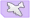轻航 稀有度 ★★☆☆☆精锐 阵营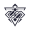传颂之物 建造时间 02:25:00（活动已关闭） 普通掉落点 活动掉落点 营养价值 炮击0 雷击0 航空29 装填11 退役收益 金币7 燃油3 勋章4 强化所需经验 炮击 0*0=0 雷击 0*0=0 航空 43*20=860 装填 43*40=1720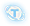+

0+
-
Lv.120+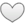耐久 C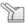防空 B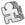机动 C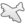航空 B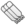雷击 E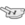炮击 E

 突破升星效果 一阶 所有轰炸机+1/战斗机效率提高5% 二阶 机库容量+1/所有战斗机+1/战斗机效率提高10% 三阶 所有舰载机+1/轰炸机效率提高10%
 初始装备 装备说明 槽位/装备类型/武器效率初始/满破/武器数满破/预装填数满破 槽 装备类型 效率 武器数 预装填数 1 战斗机 115%/130% 3 0 2 轰炸机 115%/125% 3 0 3 防空炮 80%/80% 1 0 4 设备、反潜机 - - - 5 设备、反潜机 - - - 注 水面舰艇鱼雷底座+1与航母机库+1并不增加武器数 1 - 2 - 3 -
 技能航空、装填、命中、特殊弹幕；自身增益锁之巫女 与萨拉娜同在一个舰队时，自身航空提高15.0%(35.0%)，装填、命中提高10.0%(20.0%)双影无双 与萨拉娜同在一个舰队时，战斗开始后每隔55秒，触发特殊弹幕(Lv.1（Lv.10）)，每场战斗最多触发2次(特殊弹幕威力依据技能等级)
 立绘 通常换装改造誓约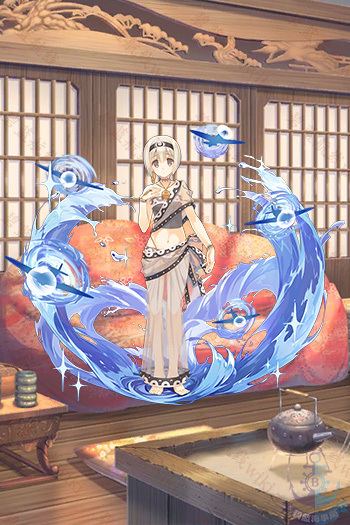请上传文件『乌璐露改造.jpg』，文件大小不超过2M。 请上传文件『乌璐露誓约.jpg』，文件大小不超过2M。 角色信息 实装 2018年11月29日 身份 锁之巫女，女仆，污女，锁之污 性格 冷淡 关键词 无口 持有物 发色 淡黄 瞳色 淡灰 萌点 双胞胎（白），白丝，独角，女仆装 CV 佐仓 绫音（さくら あやね Sakura Ayane） 推特 / 事务所简历 画师 みつみ美里+ 微博 - 推特 みつみ美里 PIXIV
 评价 双子强度中规中矩，但难有双轻航上场机会，弹幕触发点也比较晚，并不实用。 备注 技能弹幕为航空穿甲伤害，基础伤害225伤害补正200%，对甲补正一视同仁，必定暴击。

登陆界面 碧蓝航线！ 锁之巫女，乌璐露——主人的血肉人偶 乌璐露 乌璐露 早安 详细 主人不在这里…… ……她们在这里 好闲 朋友 想要晚上的侍奉？不行 严禁触碰 任务堆积 任务完成 邮件来了 欢迎回来 失望…我们，只有主人 下仆的义务，是服务指挥官大人 从早安到晚安都在一起 好感度上升中 喜欢你，指挥官大人 在此结下盟约之誓 回来了 强化成功 伟大之父保佑…… 赢了 输了…… 为了主人——力量、解放 还死不了……

皮肤描述 乌璐露。在此献上誓约之印。向主人献上永远的忠诚 早 大海，蓝天，宠爱，此乃浪漫 如您所愿 必定不负所望 叫我？ 这就是指挥官大人的爱、欲 请 辛苦了 满溢的至高幸福 没问题 请下命令

（来自@碧蓝航线_研发组）

## 其它舰娘

驱逐 • 杜威 • 艾尔温 • 卡辛改 • 唐斯改 • 格里德利 • 克雷文 • 麦考尔 • 莫里 • 弗莱彻 • 查尔斯·奥斯本 • 撒切尔 • 奥利克 • 富特 • 斯彭斯 • 拉德福特 • 杰金斯 • 尼古拉斯改 • 布什 • 黑泽伍德 • 贝奇 • 金伯利 • 马拉尼 • 斯坦利 • 斯莫利 • 哈尔西·鲍威尔 • 本森 • 拉菲改 • 贝利改 • 霍比 • 科尔克 • 西姆斯改 • 哈曼改 • 埃尔德里奇 • 库珀 • 奥马哈 • 罗利 • 里士满 • 孟菲斯 • 康克德 • 马布尔黑德 • 布鲁克林 • 菲尼克斯 • 海伦娜改 • 火奴鲁鲁 • 圣路易斯 • 小海伦娜 • 亚特兰大 • 朱诺 • 圣地亚哥改 • 小圣地亚哥 • 圣胡安 • 里诺 • 克利夫兰 • 哥伦比亚 • 蒙彼利埃 • 丹佛 • 小克利夫兰 • 伯明翰 • 克利夫兰(μ兵装) • 比洛克西 • 西雅图 • 彭萨科拉 • 盐湖城 • 北安普敦 • 芝加哥 • 休斯敦 • 波特兰改 • 印第安纳波利斯 • 阿斯托利亚 • 昆西 • 文森斯 • 明尼阿波利斯 • 威奇塔 • 巴尔的摩 • 布莱默顿 • 内华达改 • 俄克拉荷马改 • 宾夕法尼亚 • 亚利桑那 • 田纳西 • 加利福尼亚 • 科罗拉多 • 马里兰 • 西弗吉尼亚 • 北卡罗来纳 • 华盛顿 • 南达科他 • 马萨诸塞 • 阿拉巴马 • 佐治亚 • 长岛改 • 博格改 • 卡萨布兰卡 • 兰利改 • 突击者改 • 独立 • 巴丹 • 列克星敦 • 萨拉托加改 • 约克城 • 企业 • 大黄蜂 • 胡蜂 • 埃塞克斯 • 无畏 • 邦克山 • 香格里拉 • 鲦鱼 • 大青花鱼 • 棘鳍 • 蓝鳃鱼 • 女灶神
驱逐 • 女将改 • 阿卡司塔改 • 热心改 • 小猎兔犬 • 大斗犬 • 彗星改 • 新月改 • 小天鹅改 • 狐提改 • 命运女神改 • 格伦维尔 • 萤火虫 • 勇敢 • 猎人 • 标枪改 • 天后 • 丘比特 • 泽西 • 吸血鬼 • 无敌 • 火枪手 • 回声 • 爱斯基摩人 • 伊卡洛斯 • 利安得改 • 阿基里斯改 • 阿贾克斯改 • 黛朵 • 天狼星 • 黑太子 • 赫敏 • 南安普顿 • 谢菲尔德 • 纽卡斯尔改 • 谢菲尔德(μ兵装) • 格拉斯哥 • 格罗斯特 • 爱丁堡 • 贝尔法斯特 • 小贝法 • 阿瑞托莎 • 加拉蒂亚 • 欧若拉 • 斐济 • 牙买加 • 库拉索改 • 杓鹬改 • 确捷 • 海王星 • 伦敦改 • 什罗普郡 • 苏塞克斯 • 肯特 • 萨福克改 • 诺福克 • 多塞特郡 • 约克改 • 埃克塞特改 • 柴郡 • 德雷克 • 声望 • 反击 • 小声望 • 胡德 • 伊丽莎白女王 • 厌战改 • 英勇 • 纳尔逊 • 罗德尼 • 英王乔治五世 • 威尔士亲王 • 约克公爵 • 豪 • 君主 • 竞技神改 • 独角兽 • 半人马 • 追赶者 • 英仙座 • 鹰 • 皇家方舟 • 光辉 • 胜利 • 可畏 • 小光辉 • 光荣 • 黑暗界 • 恐怖 • 阿贝克隆比
驱逐 • 吹雪 • 浦波 • 绫波改 • 晓 • 响 • 雷 • 电 • 白露 • 夕立 • 时雨改 • 江风 • 雪风 • 阳炎改 • 不知火改 • 野分 • 黑潮 • 亲潮 • 浦风 • 矶风 • 滨风改 • 谷风改 • 初春改 • 若叶 • 初霜改 • 有明 • 夕暮改 • 神风改 • 松风改 • 旗风 • 睦月改 • 如月改 • 卯月 • 水无月 • 文月 • 长月 • 三日月 • 清波 • 卷波 • 长波 • 新月 • 春月 • 宵月 • 花月 • 朝潮 • 大潮 • 满潮 • 荒潮 • 霞 • 北风 • 夕张改 • 长良 • 五十铃改 • 鬼怒改 • 阿武隈改 • 最上改 • 三隈 • 川内改 • 神通改 • 那珂 • 阿贺野 • 能代 • 古鹰改 • 加古改 • 青叶 • 衣笠 • 妙高 • 那智 • 足柄 • 高雄 • 爱宕 • 摩耶 • 鸟海 • 铃谷 • 伊吹 • 金刚 • 比叡 • 榛名 • 雾岛 • 小比叡 • 天城 • 扶桑改 • 山城改 • 伊势改 • 日向改 • 长门 • 陆奥 • 加贺BB • 土佐 • 三笠 • 骏河 • 出云 • 飞鹰 • 隼鹰 • 凤翔 • 龙骧 • 龙凤 • 祥凤改 • 赤城 • 加贺 • 小赤城 • 赤城(μ兵装) • 苍龙改 • 飞龙改 • 翔鹤 • 瑞鹤 • 大凤 • 伊19 • 伊26 • 伊25 • 伊58 • 伊56 • 伊168 • 明石 • 伊13 • 吾妻
驱逐 • Z1改 • Z2 • Z18 • Z19 • Z20 • Z21 • Z23改 • Z25 • Z35 • Z36 • Z46 • 柯尼斯堡 • 卡尔斯鲁厄改 • 科隆改 • 莱比锡改 • 美因茨 • 希佩尔海军上将 • 欧根亲王 • 希佩尔海军上将(μ兵装) • 德意志 • 斯佩伯爵海军上将 • 罗恩 • 沙恩霍斯特 • 格奈森瑙 • 奥丁 • 俾斯麦 • 提尔比茨 • 腓特烈大帝 • 齐柏林伯爵 • 小齐柏林 • U-81 • U-557 • U-556 • U-47 • U-73 • U-101 • U-522 • U-110
驱逐 • 鞍山 • 抚顺 • 长春 • 太原 • 逸仙 • 宁海改 • 平海改
驱逐 • 龙骑兵 • 特伦托 • 扎拉 • 利托里奥 • 加富尔伯爵 • 朱利奥·凯撒
驱逐 • 威严 • 明斯克 • 塔什干 • 阿芙乐尔 • 水星纪念 • 恰巴耶夫 • 甘古特 • 苏维埃罗西亚
驱逐 • 凯旋 • 福尔班改 • 鲁莽 • 倔强 • 埃米尔·贝尔汀改 • 圣女贞德 • 路易九世 • 黎塞留 • 香槟 • 贝亚恩 • 絮库夫
驱逐 • 勒马尔改 • 塔尔图 • 沃克兰 • 恶毒 • 拉·加利索尼埃 • 阿尔及利亚 • 敦刻尔克 • 让·巴尔 • 加斯科涅(μ兵装) • 加斯科涅
驱逐 • 泛用型布里 • 试作型布里MKII
驱逐 • 布兰 • 群白之心 • 涅普顿 • 绀紫之心 • 诺瓦露 • 圣黑之心 • 贝露 • 翡绿之心
驱逐 • 22 • 33
驱逐 • 猫音 • 露露缇耶 • 久远 • 乌璐露 • 萨拉娜 • 芙米露露
驱逐 • 绊爱 • 绊爱·Elegant • 绊爱·SuperGamer • 绊爱·Anniversary
驱逐 • 白上吹雪 • 夏色祭 • 百鬼绫目 • 紫咲诗音 • 时乃空 • 大神澪 • 湊阿库娅